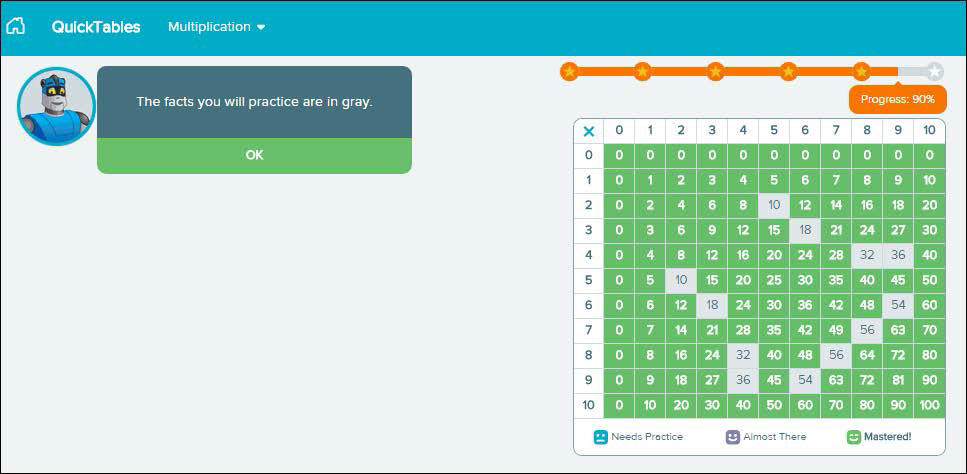# ALEKS QuickTables for Grades 3–12​

ALEKS QuickTables is a research-based, math fact mastery program for multiplication, division, addition, and subtraction for grades 3 and up. The online program offers an interactive Student Module with ongoing assessment, learning, review, and visual tracking of student progress. ​

In addition, a powerful Teacher Module allows teachers to monitor student progress, generate customized worksheets, and adjust program settings to best fit student and class needs. ALEKS QuickTables requires minimal teacher involvement for immediate implementation.​ALEKS Quicktables Courses

## Elementary School

#### 3rd Grade Arithmetic (with QuickTables) (EN-SP)

Course Curriculum:

• Whole Numbers
• Fractions
• Decimals
• Measurement and Geometry
• Other Topics Available(*)

View Full Course Curriculum

#### 4th Grade Arithmetic (with QuickTables)

Course Curriculum:

• Whole Numbers
• Fractions
• Decimals
• Measurement and Geometry
• Other Topics Available(*)

View Full Course Curriculum

#### 5th Grade Arithmetic (with QuickTables)

Course Curriculum:

• Whole Numbers
• Fractions
• Decimals
• Measurement and Geometry
• Proportions and Percents
• Signed Numbers and Exponents
• Other Topics Available(*)

View Full Course Curriculum

#### 6th Grade Arithmetic (with QuickTables)

Course Curriculum:

• Whole Numbers
• Fractions
• Decimals
• Measurement and Geometry
• Proportions and Percents
• Signed Numbers and Exponents
• Other Topics Available(*)

View Full Course Curriculum

#### Mathematics - LV 3 (with QuickTables)

Course Curriculum:

• Whole Number Place Value
• Subtracting Whole Numbers
• Multiplying Whole Numbers
• Dividing Whole Numbers
• Fractions
• Measurement
• Representing and Interpreting Data
• Perimeter and Area
• Geometry
• Other Topics Available(*)

View Full Course Curriculum

#### Mathematics - LV 4 (with QuickTables)

Course Curriculum:

• Whole Number Place Value, Addition, and Subtraction
• Multiplying Whole Numbers
• Dividing Whole Numbers
• Expressions and Equations
• Fraction Equivalence
• Operations with Fractions
• Fractions and Decimals
• Measurement
• Perimeter and Area
• Geometry
• Other Topics Available(*)

View Full Course Curriculum

#### Mathematics - LV 5 (with QuickTables)

Course Curriculum:

• Place Value and Review of Arithmetic
• Multiplying Whole Numbers
• Dividing Whole Numbers
• Multiplying and Dividing Decimals
• Fractions
• Operations with Fractions
• Expressions and Equations
• Measurement
• Geometry
• Perimeter, Area, and Volume
• Other Topics Available(*)

View Full Course Curriculum

## Middle School

#### Essential Mathematics (with QuickTables)

Course Curriculum:

• Whole Numbers
• Decimals
• Fractions
• Ratios, Proportions, and Measurement
• Percents
• Integers and Rational Numbers
• Equations and Inequalities
• Graphs and Functions
• Lines, Angles, and Polygons
• Transformations
• Perimeter, Area, and Volume
• Data Analysis and Probability
• Other Topics Available(*)

View Full Course Curriculum

*Other Topics Available:
By default, these topics are NOT included in the course, but can be added using the content editor in the Teacher Module.

QuickTables:
ALEKS QuickTables is a research−based, math fact mastery program for multiplication, division, addition, and subtraction for grades 3 and up. The online program oﬀers ongoing assessment, learning, review, and visual tracking of student progress.